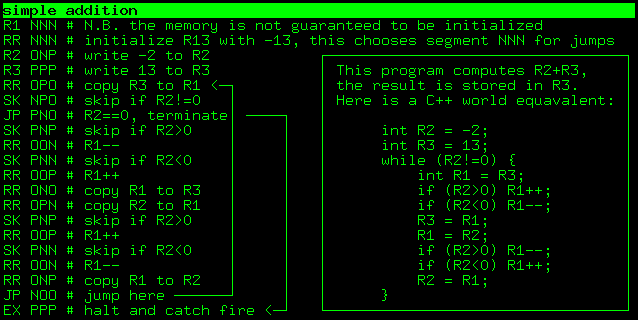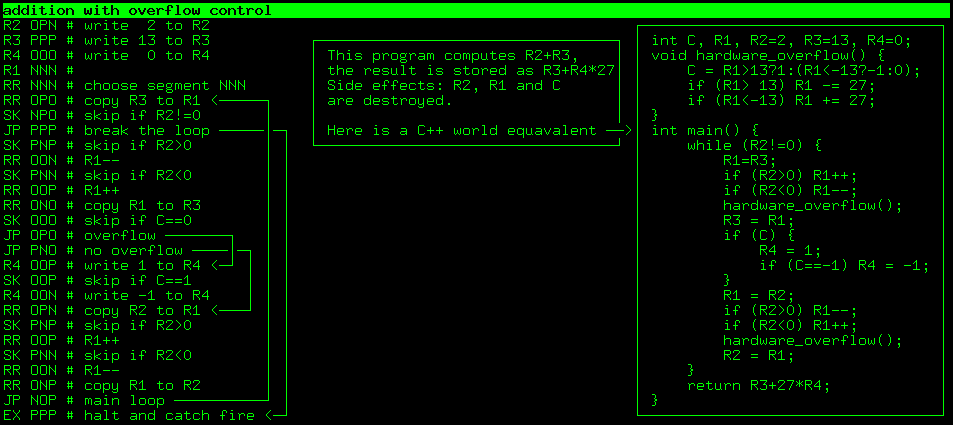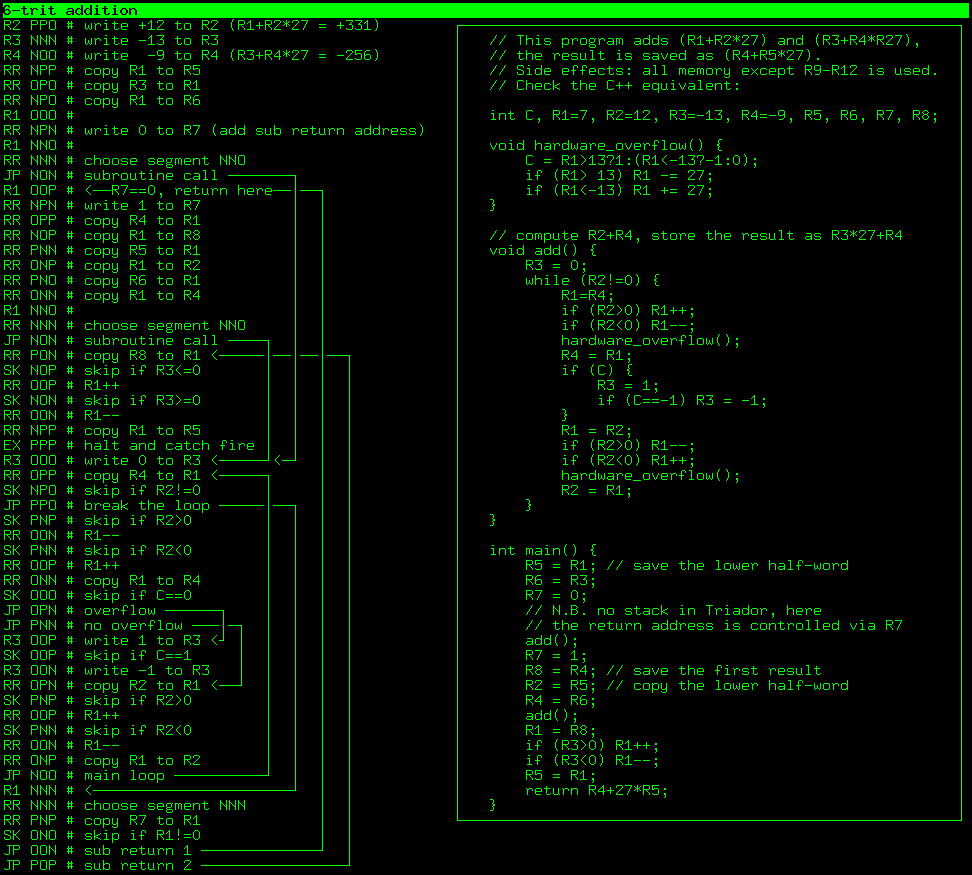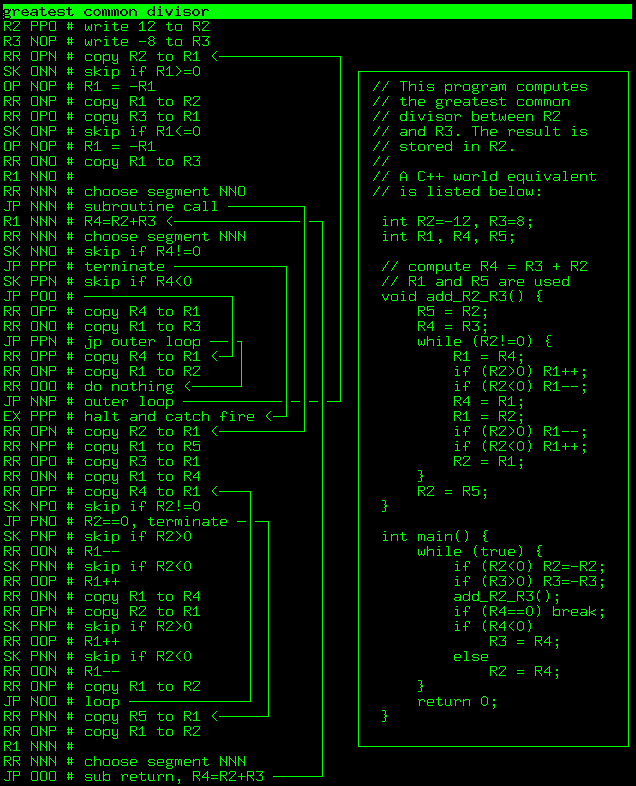Click the “chat” button below for chat support from the developer who created it, or find similar developers for support.ssloy158 Stars7 ForksDo What The F*ck You Want To Public License68 Commits1 Opened issues#### Description

Ternary computer emulator!
?

#### Need anything else?#### Contributors list# 1,277
C++
c-plus-...
Shell
raytrac...
56 commits# 653,092
C++
4 commits

# The ultimate goal: a ternary computer

Many claimed to build a ternary computer, however nobody (to the best of my knowledge) completed the project. Triador project makes no empty promises!

I am building a very simple but functional 3-trit balanced ternary computer. The only building block allowed is a ternary multiplexer based on DG403 analog switches. You can find more details on the build at the homebrew ternary computer hackaday project. Also check this youtube mini-series about the hardware:# Architecture description

This repository contains a program emulator that eases the debugging of the hardware. Triador has a 3-trit architecture, this means that its registers can store numbers from -13 to +13. It has four main registers R1-R4 and nine extra registers R5-R13. Note that R13 is a special register used to choose current program memory segment (more on this below). So, in total, Triador can store 13 numbers from the [-13..+13] range. In addition to that, it has a 1-trit borrow/carry flag and a 6-trit program counter register. The read-only program memory has 27 segments, 27 instructions per segment. So, max program size is 729 instructions. Here is a graphic description:# Instruction set

Triador has a very limited instruction set, it is very close to Brainfuck in terms of expressivity, but offers a much more readable code (check for the examples below).

Triador understands 9 instructions; each instruction has a mandatory 3-trit argument. Note that the extension instruction at the moment is interpreted as halt and catch fire. Here is the complete list of available instructions:# Interpreter compilation

```git clone https://github.com/ssloy/triador.git
mkdir build
cd build
cmake ..
make
```

You can open the project in Gitpod, a free online dev evironment for GitHub:On open, the editor will compile & run the program. Just change the code in the editor and rerun the script (use the terminal's history).

# Program file specifications

The program file must contain a single instruction per line. The instruction must be in the first 6 characters of each line, any character beyond the first 6 is discarded. Therefore, each line must contain one of the following instructions, where ttt means a 3-trit number with values from NNN (-13) to PPP (+13): * EX ttt * JP ttt * SK ttt * OP ttt * RR ttt * R1 ttt * R2 ttt * R3 ttt * R4 ttt

The emulator prints the complete state of the Triador computer for every instruction computational step.

# Example programs

Note that Triador has no input interface. To enter your data into the computer, use R1-R4 commands.

I want to sum two numbers written in registers R2 and R3. But the Triador knows nothing about addition! It only knows how to do increment/decrement. Let's start by writing a simple program in an environment you master. For a start, I assume that R2 and R3 are non-negative. We can decrement R2 and increment R3 simultaneously until R2 reaches zero:

```int main() {
unsigned int R2 = 2;
unsigned int R3 = 11;
while (R2!=0) {
R3++;
R2--;
}
return R3;
}
```

Okay, what about signed numbers? No problem, we can adapt our code a bit in a way that the absolute value of R2 decreases with each iteration:

```int main() {
int R2 = -2;
int R3 = 13;
while (R2!=0) {
if (R2>0) R3++;
if (R2<0) R3--;
if (R2>0) R2--;
if (R2<0) R2++;
}
return R3;
}
```

That is all! Here is a very simple program that writes two numbers to the registers R2 and R3 and computes their sum. The result is stored in R3:The first column (6 first characters of each line) is the actual program. The program counter (PC) is initialized to NNN NNN. Recall that any character beyond the first 6 is considered to be a comment.

Here is an execution log:

```\$ ./triador ../prog/add.txt | tail -n 3
R1  R2  R3  R4  R5  R6  R7  R8  R9 R10 R11 R12 R13  C   PC
11   0  11   5   0   6 -12  -2  11   8  11 -10 -13  0  -345
```
Note that R3 contains 11, the result of -2 + 13.

Triador does not support while loops directly, it uses unconditional jumps JP; branching is done via SK operation, which allows us to skip the next operation.

Note that the unconditional jump command JP ttt has a mandatory three-trit argument ttt, wherease the program counter PC is a six-trit number. Three missing trits come from register R13. The JP ttt command jumps to the instruction number 27 * R13 + ttt. The very first program memory segment has the number NNN (-13), and this is why my program starts with

```cpp
R1 NNN # write -13 to R1
RR NNN # copy R1 to R13
```
All the program fits into one segment, therefore after this initialization I do not modify R13, and all the jumps are performed in this segment.

This program writes two numbers to the registers R2 and R3, and computes their sum. The result is stored as R3 + R4 * 27, that is, R4 can be -1, 0 or 1 and represents an eventual overflow of R2+R3. This also means that the result is stored in a word data type R3, R4.```\$ ./triador ../prog/add-with-overflow-control.txt |tail -n 3
R1  R2  R3  R4  R5  R6  R7  R8  R9 R10 R11 R12 R13  C   PC
-12   0 -12   1  -9   2 -12  -6   4   5   6   7 -13  0  -338
```
Note that R3 + 27 * R4 is equal to 15, the result of 2+13 operation. It is easy to see that I have simply added the overflow tracking into the addition:
```cpp
[...]
SK OOO # skip if C==0
JP OPO # overflow ───────┐
JP PNO # no overflow ────│─┐
R4 OOP # write 1 to R4

Are [-13..+13] registers not expressive enough? No problems, let us continue with the word data type.
This program writes a 6-trit number to R1,R2 and a second 6-trit number to R3,R4. Then a 6-trit sum is computed and stored in R4,R5.R1  R2  R3  R4  R5  R6  R7  R8  R9 R10 R11 R12 R13  C   PC
3   0   0  -6   3 -13   1   3   6   5  13  -2 -13  0  -335

Note that R4+27 * R5 = 75, and we asked for the computer to compute 331-256. Do I have to explain how this code works? If you've figured out the previous two, I don't think it's necessary. We add the two upper half words, then we add the lower half words. If there is an overflow when summing up the lower halves, we increment/decrement the first sum. If you have troubles understanding this text, simply check the equivalent C++ code :)

There is one subtlety in this code: since I want to add three-trit numbers twice, I mimic a function call. However, Triador does not have a stack, and it knows nothing about subroutines and return addresses. No problem, I control the return address from this ersatz-subroutine via register R7:
[...]
SK ONO # skip if R1!=0
JP OON # sub return 1
JP POP # sub return 2

Greatest common divisor

Of course, our example set would not be complete without Euclid's algorithm. This program computes the greatest common divisor between R2 and R3,
the result is stored back in R2.\$ ./triador ../prog/gcd.txt |tail -n 3
R1  R2  R3  R4  R5  R6  R7  R8  R9 R10 R11 R12 R13  C   PC
-13   4  -4   0   4  12  13   1  -7  -9   3   4 -13  0  -338

Note that R2 is equal to 4, the greatest common divisor of 12 and -8.
```